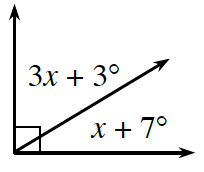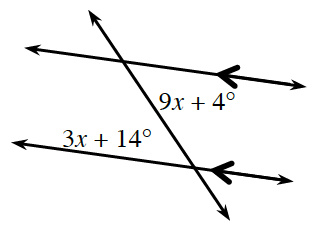### Home > CCG > Chapter 4 > Lesson 4.1.5 > Problem4-48

4-48.

For each diagram below, write an equation and solve for $x$, if possible.

1.The two angles are complementary.

1.Refer to the Math Notes box in Lesson 2.1.4.

The two angles are congruent.